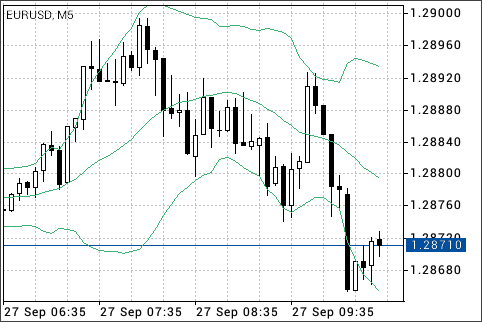# 布林带 (Bollinger Bands®)

1. 由于波动性降低带宽收缩，易于发生价格突变;
2. 如果价格突破上边带，预期当前的趋势会延续;
3. 如果尖顶和深坑超出带宽之后又缩回带宽之内，也许趋势会逆转;
4. 若价格走势始自一条边带线，那么通常会到达相对一侧。## 计算

ML = SUM (CLOSE, N) / N = SMA (CLOSE, N)

TL = ML + (D * StdDev)

BL = ML - (D * StdDev)

SUM (..., N) ― N 周期的总和;
CLOSE ― 收盘价;
N ― 计算所用的周期数;
SMA ― 简单移动均线;
SQRT ― 平方差;
StdDev ― 标准偏差:

StdDev = SQRT (SUM ((CLOSE �D SMA (CLOSE, N))^2, N)/N)